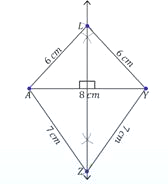## Practical geometry of Class 8

### CONSTRUCTING A QUADRILATERAL WHEN FOUR SIDES AND A DIAGONAL OF A QUADRILATERAL ARE GIVEN:

We consider the quadrilateral ABCD as a figure made of two triangles:

• ΔABC and ΔADC when diagonal AC as the common side is given.
• ΔABD and ΔBCD when diagonal BD as the common side is given.

In order to draw the quadrilateral when four sides and one diagonal are given, we first draw a rough sketch of the quadrilateral and write its dimensions along the sides and then we divide it into two triangles which can be drawn conveniently.

### STEPS:

Step 1: Construct a triangle ABD (say).

Step 2: Find point C opposite to the vertex A as follows. With B as the centre and given radius, draw an arc on the other side of BD. Similarly with D as the centre and given radius, draw another arc intersecting the previous arc. The point of intersection of these arcs is marked as C.

Step 3: join points B and C, and D and C.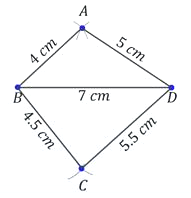question 1. Construct a quadrilateral ABCD, in which AB = 5.5 cm, AD = 4.4 cm, CD = 6.5 cm, and AC = 6.5 cm and BD = 7.1 cm.

Solution: First we draw a rough sketch of quadrilateral ABCD. It is evident from the rough sketch that we have sufficient data to draw triangles ADC and ABD.

 Draw AC = 6.5 cm.  With A as centre and radius AD = 4.4 cm, draw an arc. With C as centre and radius CD = 6.5 cm, draw an arc to intersect the arc drawn in (ii) at D. With A as centre and radius AB = 5.5 cm, draw an arc on the side of AC opposite to that of D. With D as centre and radius BD = 7.1 cm, draw another arc to intersect the arc drawn in (iv) at B.• Join AD, CD, AB and CB to obtain the required quadrilateral.

question 2. Construct a quadrilateral ABCD, when AB = 4 cm, BC = 6.0 cm, CD = DA = 5.2 cm and AC = 8 cm.

Sol. (i) Construct AC = 8 cm as shown in figure.

 With A as centre and radius AB = 4 cm, draw an arc. With C as centre and radius CB = 6.0 cm, draw another arc to cut the arc of step (ii) at B. With A as centre and radius AD = 5.2 cm, draw an arc on the opposite side of AC. With C as centre and radius CD = 5.2 cm, draw another arc to cut the arc of step (iv) at D. Join AB, BC, CD and DA.Thus, ABCD is the required quadrilateral.

question 3. Construct the quadrilateral ABCD in which AB = 4.5 cm, BC = 5.5 cm, CD = 4, cm, AD = 6 cm and AC = 7 cm.

 Solution: Steps of construction Take a line segment AC = 7 cm. Draw an arc of radius AB = 4.5 cm taking A as centre. Draw another arc of radius 5.5 cm taking C as centre. Let these two arc intersect at B. Triangle ABC is obtained.•  Repeat the construction to obtain ΔADC taking AD = 6 cm and DC = 4 cm.
•  ABCD is the required quadrilateralquestion 4. Construct the quadrilateral JUMP in which JU = 3.5 cm, UM = 4 cm, MP = 5 cm, PJ = 4.5 cm and PU = 4 cm.

 Solution: Steps of construction Draw a line segment PU = 4 cm. Taking P as centre cut off an arc of radius 4.5 cm. Taking U as centre cut off another arc of radius 3.5 cm. Let these two arcs intersect at J. Join PJ and UJ. Similarly, obtain the point M on the opposite side of diagonal PU. Hence, JUMP is the required quadrilateral.question 5. Construct a parallelogram ABCD in which, AB = 6 cm, BC = 4.5 cm and diagonal AC = 6.8 cm.

 Solution: Steps of construction Draw AB = 6 cm. With A as centre and radius 6.8 cm draw an arc. With B as centre and radius 4.5 cm draw another arc, so as it cuts the previous arc of step (ii) at C.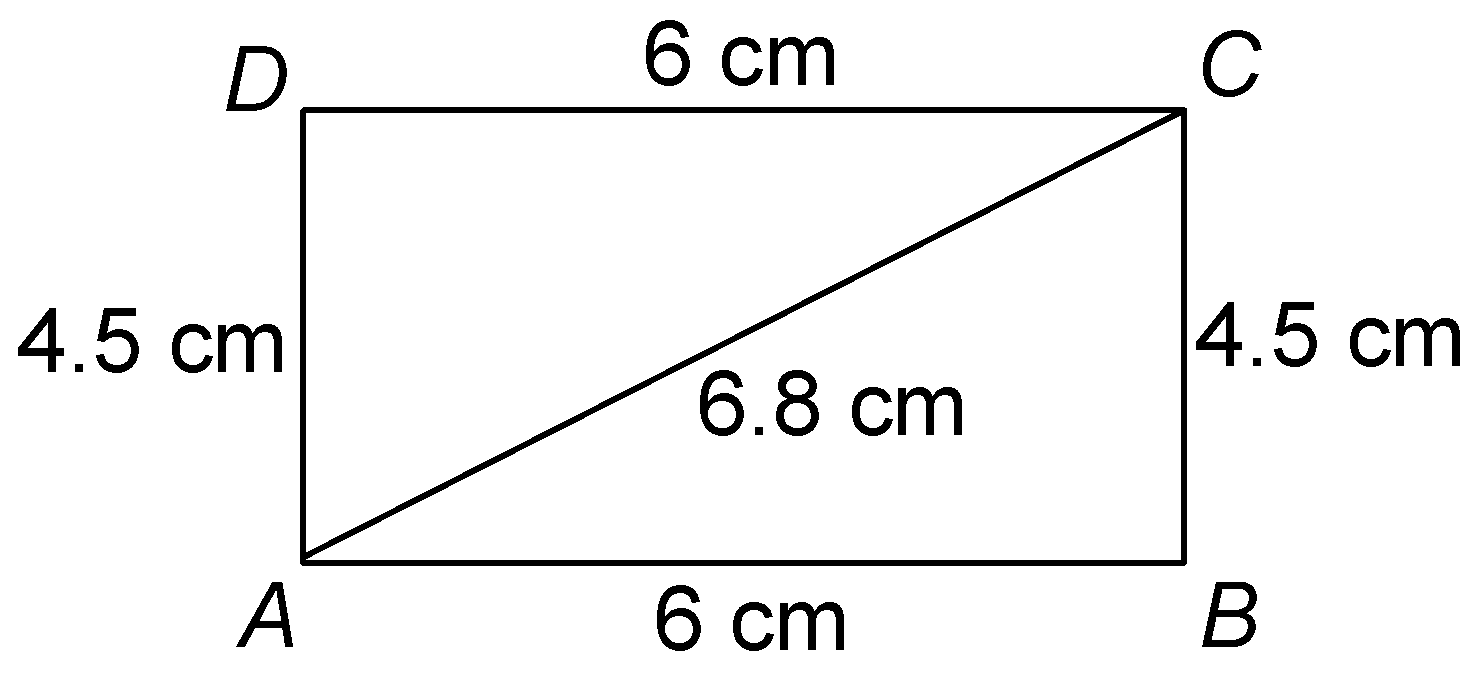•  Join BC and AC.
• With A as centre and radius 4.5 draw an arc.
• With C as centre and radius 6 cm draw another arc so as it cuts the previously drawn arc of step (v) at D.
• Join DA and DC.

Then, ABCD is the required parallelogram.

### CONSTRUCTING A QUADRILATERAL WHEN LENGTHS OF ITS THREE SIDES AND TWO INCLUDED ANGLES:

STEPS:
Step 1: Draw a line segment MN (say) of given length.

Step 2: Construct a given angle at M.

Step 3: Construct an angle 90° at N.

Step 4: Locate vertices L and O.

Step 5: Join L and O.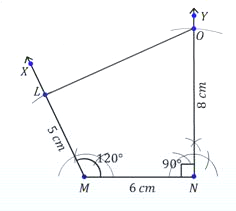question 1. Construct a quadrilateral ADBC, in which AB = 4.4 cm, BC = 4 cm,  CD = 6.4 cm, DA = 2.8 cm, and BD = 6.6 cm. Measure the length of AC.

 Solution: Steps of construction Draw a line segment AB = 4.4 cm. With centre A and radius 2.8 cm, draw an arc. Then, with centre B and radius 6.6 cm, draw another arc which cuts the earlier arc of this step at D.  Join AD and BD.• With centre B and radius 4 cm, draw an arc. Then, with centre D and radius 6.4 cm, draw another arc which cuts the earlier arc of this step at the point C.
• Join BC and DC.

Thus, ABCD is the required quadrilateral.

Join AC. Length of AC = 6 cm.

question 2. Construct a quadrilateral ABCD in which BC = 4 cm, CA = 5.6 cm, AD = 4.5 cm, CD = 5 cm, and BD = 6.5 cm.

 Solution:Steps of construction Draw a line segment CD = 5 cm. With centre D and radius 4.5 cm, drawn an arc. Then, with centre C and radius 5.6 cm, draw another arc. Which cuts the earlier arc of this step at A.• Join DA and CA.
• With centre D and radius 6.5 cm, draw an arc. Then with centre C, and radius 4 cm, draw another arc which cuts the earlier arc of this step at point B.
• Join CB and B.

Thus, ABCD is the required quadrilateral.

question 3. Construct the quadrilateral LIFT in which LI = 4 cm, IF = 3 cm, TL = 2.5 cm, LF = 4.5 cm and IT = 4 cm.

Solution:Steps of construction

• Take a line segment LF = 4.5 cm
 With centre as L and F cut off arcs of radii 4 cm and 3 cm respectively. Triangle LFI is obtained. Now, with L and I as centre cut off arcs of length TL = 2.5 cm and TI = 4 cm. Join TI, TL and TF. LIFT is the required quadrilateral.question 4. Construct the quadrilateral GOLD in which OL = 7.5 cm, GL = 6 cm, GD = 6 cm, LD = 5 cm and OD = 10 cm.

 Solution: Here, GL = 6 cm   GD = 6 cm and   LD = 5 cm. Steps of construction Take a line segment GL = 6 cm. Cut off GD = 6 cm and LD = 5 cm. Join GD and LD. Triangle GLD is obtained.• Now, with L and D as centre cut off two arcs of length 7.5 cm and 10 cm respectively to obtain a point O.
• Join OL, OD and OG.
•  Required quadrilateral GOLD is obtained.### CONSTRUCTING A QUADRILATERAL WHEN LENGTHS OF ITS ADJACENT SIDES AND THREE ANGLES ARE GIVEN:

Step 1: Draw a line segment of EF (say) of given length.

Step 2: Construct a given angle at E.

Step 3: Construct a given angle at F.

Step 4: Locate point G.

Step 5: Locate point H.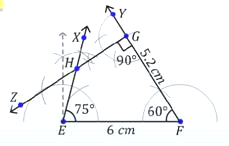question 1. Construct the quadrilateral MORE in which MO = 6 cm, OR = 4.5 cm, ∠M = 60º, ∠O = 105º, ∠R = 105º.

Solution:Steps of construction Take a line segment MO = 6 cm. Construct ∠MOR = 105º and cut off OR = 4.5 cm. At R construct ∠ORX = 105º. Now, construct ∠OMY = 60º. Let MY intersect RX at E. Thus, MORE is the required quadrilateral.question 2. Construct a rectangle OKAY in which OK = 7 cm and KA = 5 cm.

Solution:Steps of construction Draw a line segment OK = 7 cm. Construct ∠OKX = 90º and cut off KA = 5 cm. Also, construct ∠KOZ = 90º and cut off OY = 5 cm. Join OY and AY. Thus, OKAY is the required rectangle.question 3. Construct a quadrilateral ABCD in which, AB = 4.3 cm, BC = 5 cm, ∠A = 60°, ∠B = 100° and ∠C = 125°.

 Solution: Steps of construction Draw AB = 4.3 cm. Make ∠ABX = 100°. With B as centre and radius 5 cm, draw an arc, so as it cuts BX at C. Make ∠BCY = 125° and ∠BAZ = 60°. Extend, so as CY and AZ cuts at D.Then, ABCD is the required quadrilateral.

question 4. (i) Can a quadrilateral PLAN be constructed if PL = 6 cm, LA = 9.5 cm,      ∠P = 75º, ∠L = 150º and ∠A = 140º.

(ii) In a parallelogram, the lengths of adjacent sides are known. Do we still need measures of the angles to construct the parallelogram?

Solution: (i) ∠P + ∠L + ∠A = 365º

75º + 150º + 140º = 365º

Sum of four angles in a quadrilateral is 360º.

∴ Quadrilateral is not possible to construct.

(ii) No, it is not required to have the measure of angles because opposite sides of a parallelogram are equal.

If AB and BC are given

then, AB = DC, BC = AD.

## CONSTRUCTING A QUADRILATERAL WHEN LENGTHS OF ITS THREE SIDES AND TWO DIAGONALS ARE GIVEN:

STEPS:

Step1: Draw a rough sketch of the quadrilateral PQRS.

 Step 2: Draw a line segment of given length. Step 3: Construct the two given angles at two ends of the line. Step 4: With centre O and radius of the given length, draw an arc. Step 5: With centre R and radius of thje second side, draw another arc. Step 6:  Join the two arcs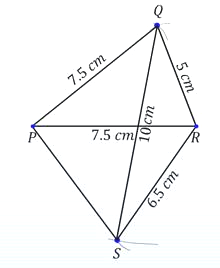question 1. Construct a quadrilateral PQRS in which PQ = 3.5 cm, QR = 2.5 cm, RS = 4.1 cm, ∠Q = 75° and ∠R = 120°. Measure side PS.

Solution:Steps of construction

•  Draw a rough sketch of the quadrilateral PQRS.
•
•
 Draw a line segment QR = 2.5 cm. Draw ∠XQR = 75° and ∠YRQ = 120°. With centre Q and radius 3.5 cm, draw an arc to cut QX at P. With centre R and radius 4.1 cm, draw an arc to cut RY at S.• Join PS.

Thus, PQRS is the required quadrilateral.

Measuring PS we find that PS = 3.7 cm.

question 2. Construct a quadrilateral ABCD in which, AB = 4.2 cm, BC = 5 cm, CD = 5.3 cm, ∠B = 120° and ∠C = 75°.

 Solution: Steps of construction Draw BC = 5 cm. Make ∠CBX = 120°. With B as centre and radius 4.2 cm draw an arc BA = 4.2 cm, so as it cuts BX at A. Make ∠BCY = 75°. With C as centre and radius 5.3 cm draw an arc so as it cuts CY at D.•  Join DA.

Thus, ABCD is the required quadrilateral.

question 3.  Construct a quadrilateral ABCD given AB = 5.1 cm, AD = 4 cm, BC = 2.5 cm, ∠A = 60° and ∠B = 85°.

Sol. Steps of construction

 Draw AB = 5.1 cm. Construct ∠XAB = 60° at A. With A as centre and radius AD = 4 cm, cut off AD = 4 cm along AX. Construct ∠ABY = 85° at B. With B as centre and radius BC = 2.5 cm, cut off BC = 2.5 cm along BY.• Join CD.

question 4. (i) Can a quadrilateral ABCD be constructed with AB = 5 cm, BC = 5.5 cm, CD = 4 cm, AD = 6 cm and ∠B = 80º?

(ii) Can a quadrilateral PQRS be constructed with PQ = 4.5 cm, ∠P = 70º,   ∠Q = 100º, ∠R = 80º and ∠S = 110º?

Solution:(i)We can construct a quadrilateral ABCD with four sides and one angle.

(ii)Here, at least one side RQ or PS is required. So, data is in-sufficient. Construction is possible only if

(a) two angles and two sides, e.g. if ∠A = 90º, ∠B = 90º, AD = 6 cm, BC = 5 cm. are given.

(b) four angles and two sides e.g. PQ = 4.5 cm, ∠P = 65º, ∠Q = 110º,              ∠QR = 4.7 cm ∠R = 115º are given.

### PARALLELOGRAM

• Opposite sides are equal.
• Opposite angles are equal.
• Sum of adjacent angles is 180º.
• Diagonals bisect each other.

### SQUARE

• All sides are equal.
• Each angle 90º.
• Diagonals are equal.

### RECTANGLE• Opposite sides are equal.
• Diagonals are equal.
• Each angle 90º.

### RHOMBUS

• Diagonals bisect each other at 90º.
• All sides are equal.
• Diagonals are unequal.

STEPS:

Step 1: Draw a side of given leng

Step 3: Draw side CE (say) of length th (say) CL

Step 2: Draw side LU (say) of given length perpendicular to CL at L. equal to LU and perpendicular to CL at C.

Step 4: Draw side UE.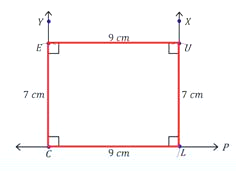STEPS:

Step 1: Draw diagonal (say) AY and its perpendicular bisector.

Step 2:  Draw sides say AL and AZ of given length.

Step 3: Draw sides LY and YZ.